Study Guide

# Slope of a Line

## Slope of a Line

What does the word "slope" make you think of? We start thinking about skiing down the Appalachians in a snazzy snowsuit and mirrored goggles. We're carving up the mountain, riding a wave of soft powder to the bottom, high-fiving mountain lions and bears as we pass them by. Good times.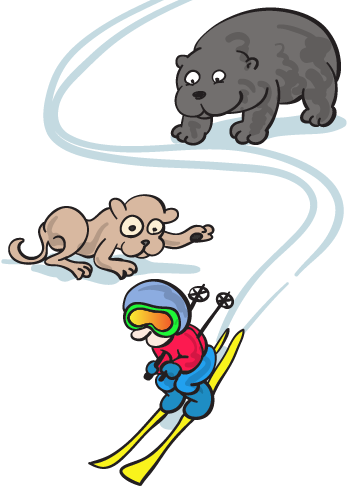The second thing we start thinking about is lines and graphing. The slope of a line measures the line’s steepness, just like the slope of a mountain measures its steepness. Now we're picturing ourselves skiing down the graph of a function.

• ### Slope

Whether we're climbing up or speeding down a mountain, we have a keen interest in its slope. It's the same way with lines, except there's a much lower chance of breaking every bone in our bodies.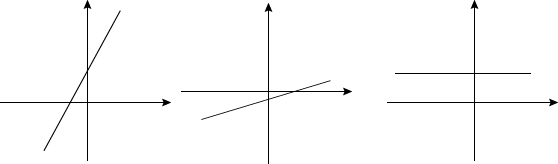When a mountain is really steep, we'd have to practically climb up it. A shallow hill, though, is easy to walk up. A flat floor is even easier than that. To find the slope, we look at the ratio of how quickly a line rises (vertically) compared to how quickly it runs (side to side).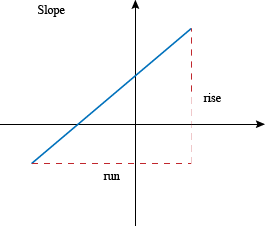### Sample Problem

Check out this graph.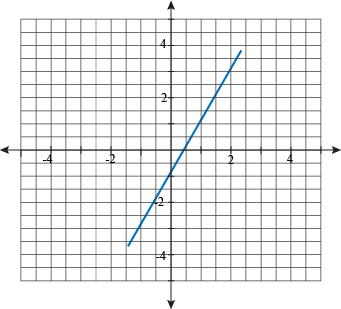If this were a mountain, it would be steep. Just how steep, though, is something we want to figure out. Well, maybe "want" is too strong of a word; more like, "feel an obligation to."

Let's pick two points on this line that we can deal with easily. Nice, whole numbers if we can get them.

The y-intercept is a good place to start, and we can see from the graph that it's at (0, -1). Another point on the line that we can cope with is (1, 1).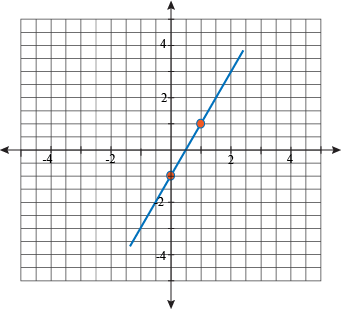The slope will equal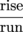. This is often said out loud as "rise over run." The "rise" is the change in vertical distance, and the "run" is the change in horizontal distance. In math, as in real life, trying to run down the mountain is a Bad Idea.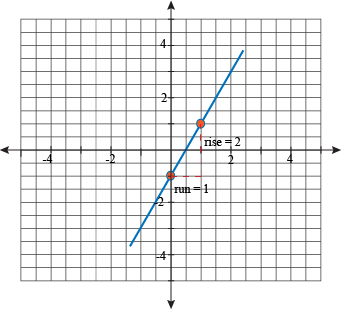When we count this out on our graph, we get a rise of 2 units and a run of 1 unit. In other words, our line moves 2 units upward every time it moves 1 unit to the right.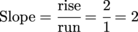Our slope is 2. It's a positive number, so we rise up and run to the right. Or, if we want to be contrary, both the rise and run could be negative, moving down and to the left. It's cool either way.

It doesn't matter what two points we chose when finding the slope; the ratio of rise over run is the same for the entire line. We're usually not happy to hear that our choices don't matter, but this time we're okay with it.

### Formulating a Slope of Attack

What if we don't want to graph a line to find its slope? Maybe there are a lot of fractions or decimals involved. Maybe our math teacher has a "no coordinate planes allowed" sign above his desk. We don't get what his problem with graphing is, but he still wants us to find the slope, and pronto.

The formula for the slope comes directly from the idea of the slope equaling rise over run. We start off with two points, (x1, y1) and (x2, y2).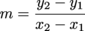Don't let the lowercase m in the formula fool you. That's the slope's code name while palling around with x and y.

A line is "rising" because its value for y is going up or down. It "runs" by changing its x-value, by going right or left. That's all this formula is doing: calculating the change in y (the rise) over the change in x (the run) from one point to another.

### Sample Problem

Find the slope of the line that passes through (0, 2) and (1, -4).

We have a new formula, and we're hankering to use it.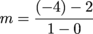Be sure to keep the x's and y's straightened out while plugging them into the formula; x1 and y1 are a couple, just like x2 and y2. It would be scandalous to take someone else's partner out on a date. Keep them together on the same side of the subtraction sign.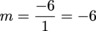This time we have a negative slope. What does that look like? Our math teacher just left the room, so let's plot our two points and see what the line looks like.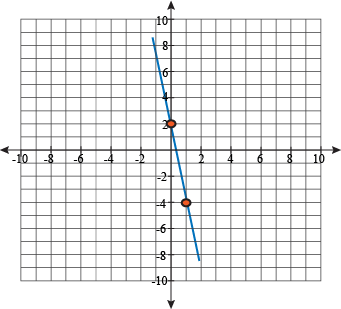We have a steep slope that goes down when moving from left to right. This downhill motion is common to all negative slopes.

Slopes don't have to be positive or negative, though.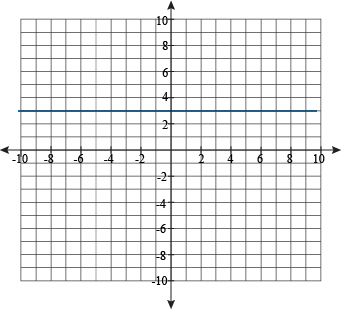A horizontal line has a rise of 0, so its slope is 0 too. Easiest mountain climb ever.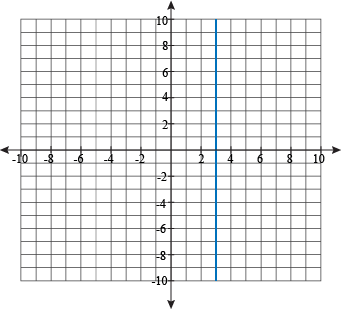A vertical line has a run of 0. From the formula, that would give us division by 0, which our teacher thinks is even worse than graphing.

So what is the slope? Just like with division by 0, any answer we try to give wouldn't make any sense. Instead, we throw up our hands and say that the slope is "undefined."

It's a good thing, too. Can you imagine trying to climb a mountain that looked like that? No thanks; we'll stay home instead.

Now quick, hide the graphs before he comes back and makes us do word problems after class.

• ### Parallel and Perpendicular Lines

Two lines walk into a bar. They order the same drinks, dance the same dances, but end up leaving without having ever met. That's the story of parallel lines.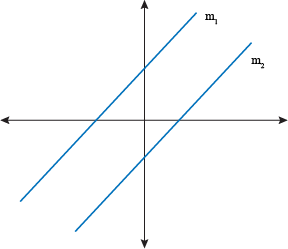Parallel lines will never intersect. This is because they share the same slope. If one line rises by 1, so does the other. A run of -3 is perfectly matched. We could watch this all day.

### Sample Problem

Show that the lines passing through these points are parallel.

(0, 5) and (2, -1)

(-1, 5) and (1, -1)

Parallel lines have never met before now, and will never meet in the future. How can we possibly predict the future of these two lines? Our crystal ball is busted, so we'll resort to showing that they have the same slope, instead.

The formula for the slope is:We'll just plug in the points from the first line into the equation. It doesn't matter which one we call point 1 and which one is point 2, as long as we stay consistent. It's like the old saying goes: you can pick your points, and you can pick your friends, but you can't pick your friends' points.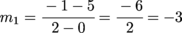Now we can find the slope of the second line. Let's do (-1, 5) for the second point, the one being subtracted.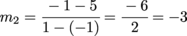Two lines with the same slope? That's parallel lines in a nutshell. Except we don't think that they'd fit in a nutshell. They'd have to meet at some point in there, right?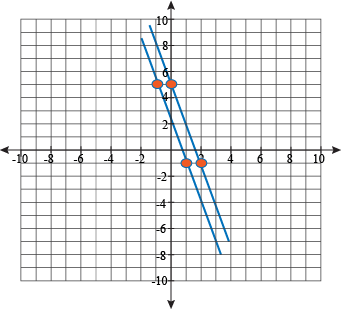The graph of the two lines shows the same thing. They share a slope, they never meet, and they are definitely not inside a nutshell.

A different relationship between two lines is when they intersect so hard that they can't intersect any harder.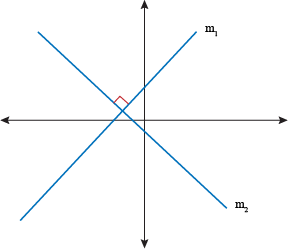These are perpendicular lines. They meet at a 90-degree angle to each other. Even so, they are total opposites of each other. Like, partners in a buddy cop movie kinds of opposites.Perpendicular lines have slopes that are the negative reciprocals of each other. Like this: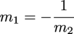It's not just the negative slope, but the negative upside-down slope.

### Sample Problem

Show that the lines passing through these points are perpendicular.

(-1, 7) and (1, -5)

(-6, 0) and (12, 3)

Once again, the key to solving this problem is to find the slopes of both lines. It's a good thing we're so pro at this.Plugging in points from the first line: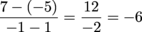One slope down, one more to go. Or should we say, one slope down and one up.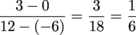Yep, -6 and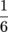are negative reciprocals. We can double-check this by multiplying them together: their product should equal -1, and it does.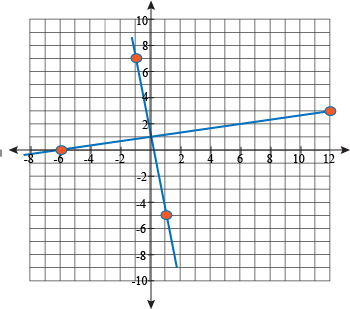We can double-check our double-check by graphing as well. The graph agrees with us, too. We love it when a plan comes together.